# 0708-1300/Class notes for Tuesday, March 18

(diff) ← Older revision | Latest revision (diff) | Newer revision → (diff)

## Typed Notes

### First Hour

Recall we had defined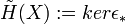$\tilde{H}(X):= ker\epsilon_*$ where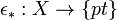$\epsilon_*:X\rightarrow\{pt\}$,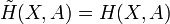$\tilde{H}(X,A) = H(X,A)$

For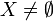$X\neq\empty$,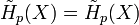$\tilde{H}_p(X) = \tilde{H}_p(X)$ for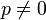$p\neq 0$ and equals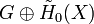$G\oplus\tilde{H}_0(X)$ for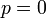$p=0$

This homology definition satisfies the axioms with the following changes: Exactness only for$X\neq\empty$ and the dimension axiom being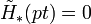$\tilde{H}_*(pt) = 0$. Furthermore, instead of additivity we have, under mild conditions of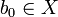$b_0\in X$ and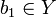$b_1\in Y$ (ie non empty) define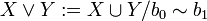$X\vee Y:= X\cup Y / b_0\sim b_1$ for a disjoint union. Then,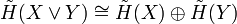$\tilde{H}(X\vee Y)\cong \tilde{H}(X)\oplus \tilde{H}(Y)$

We can actually get the above isomorphism in the following way. There are natural projection maps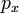$p_x$ and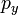$p_y$ from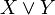$X\vee Y$ to X and Y respectively that simply contract Y and X respectively to the glued base point. There are also natural inclusion maps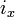$i_x$ and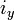$i_y$ going the other way. Then,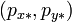$(p_{x*},p_{y*})$ and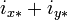$i_{x*}+i_{y*}$ are the two maps in the isomorphism. Proving they are in fact an isomorphism is a homework problem that uses excision to prove it.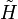$\tilde{H}$ is "kinda" natural:

We have a chain complex where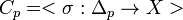$C_p = <\sigma:\Delta_p\rightarrow X>$ where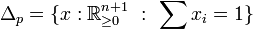$\Delta_p=\{x:\mathbb{R}^{n+1}_{\geq 0}\ :\ \sum x_i = 1\}$

We thus get that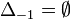$\Delta_{-1} = \empty$ since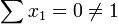$\sum x_1 = 0\neq 1$ vacuously.

Therefore,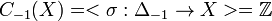$C_{-1}(X) = <\sigma:\Delta_{-1}\rightarrow X> = \mathbb{Z}$

So,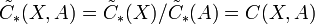$\tilde{C}_*(X,A) = \tilde{C}_*(X)/\tilde{C}_*(A) = C(X,A)$

Note: We have never actually specified that p is positive axiomatically. In fact,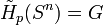$\tilde{H}_p(S^n) = G$ for p=n and 0 for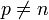$p\neq n$ works fine for all p's. So, since the spaces we are going to be interested in are those that can be constructed from spheres we really will only encounter non trivial homologies for positive p.

Degrees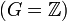$(G=\mathbb{Z})$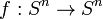$f:S^n\rightarrow S^n$ then get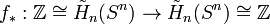$f_*:\mathbb{Z}\cong \tilde{H}_n(S^n)\rightarrow \tilde{H}_n(S^n)\cong\mathbb{Z}$

We thus define: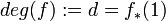$deg(f):=d = f_*(1)$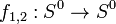$f_{1,2}:S^0\rightarrow S^0$ has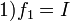$1) f_1 = I$

2)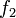$f_2$ = flip, ie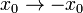$x_0\rightarrow -x_0$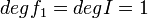$deg f_1 = deg I = 1$ in all dimensions

deg$f_2$ = deg flip = -1

Proposition

Let$f:S^n\rightarrow S^n$ be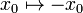$x_0\mapsto-x_0$ and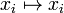$x_i\mapsto x_i$ for i>0

then def f= -1

Proof:

We get two rows of the following sequence, with the induced maps from f going vertically between them: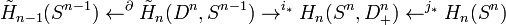$\tilde{H}_{n-1}(S^{n-1})\leftarrow^{\partial}\tilde{H}_n(D^n,S^{n-1})\rightarrow^{i_*} H_n(S^n, D^n_+)\leftarrow^{j_*}H_n(S^n)$

The resulting diagram from the two rows of the above sequence and the maps induced by f between them in fact commute at all places, where the left square commutes as a result of the properties of the connecting homomorphism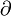$\partial$

Propositions:

1) if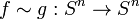$f\sim g: S^n\rightarrow S^n$ then deg f = deg g

2)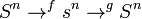$S^n\rightarrow^f s^n\rightarrow^g S^n$ then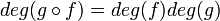$deg (g\circ f) = deg (f) deg (g)$

3) deg a where a is the antipodal map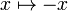$x\mapsto -x$ has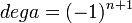$deg a = (-1)^{n+1}$ on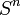$S^n$

### Second Hour

Corollary

If n is even, a is not homotopic to I

Corollary Every f:S^2\rightarrow S^2 has a fixed point, or an antipodal point. Ie. f(x) = x or f(x) = -x for some value or x. (Note this is believe true for 2n not just 2, but the follow proof appears needs some modification to work in dimensions 2n)

Proof

Suppose f has no fixed points. Thus x and f(x) are distinct and define a great circle. Thus there is a shortest path from f(x) to -x. This uniquely defines a homotopy between f and a. Suppose f also had no antipodal points. Then the same great circle defines a unique homotopy between f and I. But I is not homotopic to a, a contradiction. Q.E.D

Corollary

Every vector field on S^{2n} has a zero, i.e., "on earth there must be a windless points" or "you can't comb the hair on a coconut"

Proof A non zero vector field induces a homotopy of I to a which is impossible.

Theorem

If f:S^n\rightarrow S^n \ni y_0 is smooth (and every map may be approximated by one) and y_0\in S^n is a regular value (which occurs almost everywhere by Sard's Theorem) and f^{-1}(y_0) = \{x_1,\cdots, x_n} then deg f = \sum_{j=1}^k \pm 1 = \sum sign (det)(df_{x_i}))

.ie. we get +1 if it preserves orientation and -1 if it reverses it. The latter term is done using an identification of the coordinates near x_i w coordinates near y+0 using an orientation preserving rotation of S^n

Examples

1) S^1\rightarrow S^1 via z\mapsto z^k

This map wraps the circle around itself k times yielding k preimages for each point in the image, all with the same sign.

So, deg f = \sum_k +1 = k

2) Consider the map of a sphere where you place a plastic bag over a sphere, collect the bag at a pole, twist it once, rewrap the sphere, twist and rewrap again k times. Then the deg f = +1 -1 +1-1\cdots = 0

Proof of Theorem

1) Let T:\mathbb{R}^{n+1}\rightarrow\mathbb{R}^{n+1} be linear and norm preserving, T\in M_{n+1\times n+1} and T^{T}T = I. Then, deg T = det T

Proof: Every rigid rotation is a product of reflections.

2) Let A:\mathbb{R}^N\rightarrow\mathbb{R}^n be any n\times n non singular matrix so that A(\infty)=\infty so this induces a map \tilde{A}:S^n\rightarrow S^n. Then, deg\tilde{A} = sign(det A)

Proof: Gaussian elimination results in making A a product of "elementary matrices" which come in three types: A matrix with 1's along the diagonal except one diagonal entry being \lambda. A matrix which is the identity only with two rows interchanged. A matrix with is the identity with a \lambda in some non diagonal location.

The latter of these is clearly homotopic to the identity by simply turning the \lambda off.

The middle of these is just a reflection. The former of these, if \lambda>0 it is clearly homotopic to the identity. But if \lambda<0 then it is homotopic to a reflection.

3) f:\mathbb{R}^n\rightarrow\mathbb{R}^n such that f^{-1}(0) = 0, df|_0 = A non singular, f(\infty) = \infty so f defines \tilde{f}:S^n\rightarrow S^n then deg f = sign(det df|_0)

Proof: Consider for t\geq 1, f_t(x) := tf(x/1). Then, f_1 = f, f_{\infty}=A. This is a homotopy as it makes good sense for t\in[0,\infty]. So, deg f = deg\tilde{A}

4) All that remains to prove the theorem is the shift from (0,0) to (x_0,y_0) which induces a rotation at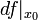$df|_{x_0}$